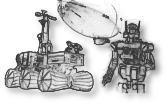## math/ros-angles

ROS simple math utilities to work with angles
Directory:
math/ros-angles (package's history)
Package version:
ros-angles-1.9.11
http://ros.org/wiki/angles
modified-bsd
Source archive:
https://github.com/ros/angles/archive/1.9.11.tar.gz
Description:
This package provides a set of simple math utilities to work with angles. The utilities cover simple things like normalizing an angle and conversion between degrees and radians. But even if you're trying to calculate things like the shortest angular distance between two joint space positions of your robot, but the joint motion is constrained by joint limits, this package is what you need.
Run dependencies:
googletest, libstdc++, py27-catkin-pkg>=0.2, py27-empy>=3, py27-nose>=0.10, py27-pyparsing>=1, py27-ros-catkin>=0.7<0.8
Build dependencies:
cmake>=2.8.3, digest>=20080510, g++>=3, gcc>=3, pax, pkg-config>=0.22, pkg_install>=20110805.12, python27>=2.7<2.8, tnftp>=20130505~ssl
General options:
debug
Produce debugging information for binary programs
c++-compiler alternatives:
g++
Use the GNU C++ compiler
clang++
Use the LLVM C++ compiler
ccache-g++
Use ccache and the GNU C++ compiler
ccache-clang++
Use ccache and the LLVM C++ compiler
c-compiler alternatives:
gcc
Use the GNU C compiler
clang
Use the LLVM C compiler
ccache-gcc
Use ccache and the GNU C compiler
ccache-clang
Use ccache and the LLVM C compiler
python alternatives:
python27
Use python-2.7
python36
Use python-3.6
python37
Use python-3.7
python38
Use python-3.8
python39
Use python-3.9
python310
Use python-3.10
python311
Use python-3.11
ros alternatives:
melodic
Use ros melodic